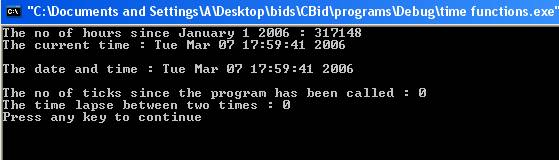Date and Time Functions

There are several functions that deal with time and date.  In order to perform these functions header file <ctime> is included into the program. The header file defines time related types such as clock_t, time_t and structure tm. The clock_t and time_t represent system time and date in the form of integer. The structure tm has data and time broken into elements. Some of the functions are:-

• asctime() - The function asctime() returns the pointer to the string containing the date and time in readable format.

• clock() - The function clock() returns the no of ticks since the calling program has been running. In order to convert no of ticks into seconds divide it by macro CLOCKS_PER_SEC which is defines in the header file <ctime>.

• ctime() - The function ctime() converts the value of time_t to string.

• difftime()-The function difftime() returns the difference in seconds between two times. It takes two arguments.

• gmtime() - The function gmtime() returns a pointer to the broken down form of the time which is in UTC(Coordinated Universal Time) format. It converts time_t to tm structure as UTC time. If the system does not support UTC a null value is returned.

• localtime()- The function localtime() returns a pointer to the broken down form of time_t into the form of tm structure as local time.

• mktime() - The function mktime() converts tm structure to time_t.

• time() - The function time() returns the current time. The function can be called using a NULL pointer or pointer to a variable of type time_t.

Here is a program which illustrates the working of time and date functions.

#include<iostream>

#include<ctime>

using namespace std;

int main()

{

time_t time1;

time_t start1,end1,start2;

struct tm * currenttime;

time(&start1);

currenttime=localtime(&start1);

time1=time(NULL);

float a=(clock());

time(&start2);

cout << "The no of hours since January 1 2006 : " << (time1/3600) << endl;

cout << "The current time : " << ctime(&time1) << endl;

cout << "The date and time : " << asctime(currenttime) << endl;

cout << "The no of ticks since the program has been called : " << (clock()) << endl;

time(&end1);

cout << "The time lapse between two times : " << difftime(end1,start2) << endl;

return(0);

}

The result of the program is:-The statement

#include<ctime>

includes a header file <ctime>  into the program. The statements

time_t time1;

time_t start1,end1,start2;

declares variables time1,start1,end1 and start2 of type time_2. The statement

struct tm * currenttime;

declares a pointer to the variable currentime of type tm structure. The statement

time(&start1);

returns the current time of the system. The statement

currenttime=localtime(&start1);

returns the localtime of the start1 into the form of tm structure. The statement

time1=time(NULL);

returns the current time of the system. The statement

cout << "The no of hours since January 1 2006 : " << (time1/3600) << endl;

displays the no of hours since January 1. The time1 is divided no of seconds in an hour. The statement

cout << "The current time : " << ctime(&time1) << endl;

displays the string format of the current time. The statement

cout << "The date and time : " << asctime(currenttime) << endl;

displays the current time and date in readable format. The statement

cout << "The no of ticks since the program has been called : " << (clock()) << endl;

prints the no of ticks since the program has been called. The statement

cout << "The time lapse between two times : " << difftime(end1,start2) << endl;

prints the time difference between end1 and start2.

Go to the previous lesson or proceed to the next lesson